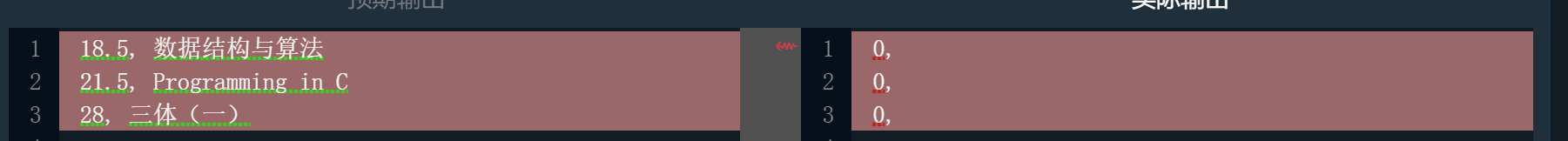2020-04-30 14:47

# 整行读入字符串到结构数组中元素的字符数组里

1. 问题要求：编写一个完整的程序，对输入的n（n<100）本书进行排序并输出。
2. 程序输入：程序首先输入一个正整数n，然后输入n本书的信息，每本书用两行输入，第一行输入书的名称（书名最长不超过50个字符，中间可能有空格）第二行输入价格，价格为浮点数。
3. 程序输出：程序要求对n本书按价格从低到高排序，如果价格相同，则按书名（字符串）字典序从小到大排序。最后输出排序结果，每行输出一本书的信息，首先输出价格，然后输出书名，中间用一个英文逗号和一个空格分开。
``````#include <string.h>
#include <iostream>

using namespace std;
struct BOOK{
char name;
float price;
};
void sort(BOOK a[],int n)
{
BOOK tmp;
for(int i=0;i<n-1;i++)
{
for(int j=0;j<n-2;j++)
{
if((a[j].price)>(a[j+1].price))
{
tmp=a[j];
a[j]=a[j+1];
a[j+1]=tmp;
}
if((a[j].price)==(a[j+1].price))
{
int flag=strcmp(a[j].name,a[j+1].name);
if(flag>0)
{
tmp=a[j];
a[j]=a[j+1];
a[j+1]=tmp;
}
}
}
}
}
//请在此添加代码，实现书籍数据的输入、排序和输出
/********** Begin *********/
int main()
{
int n;//n-the number of book
cin>>n;
BOOK a;
for(int i=0;i<n;i++)
{
cin.getline(a[i].name,51);
cin>>a[i].price;
}
sort(a,n);
for(int i=0;i<n;i++)
{
cout << a[i].price << ", "<< a[i].name <<endl;
}
return 0;
}
/********** End **********/

``````• 点赞
• 写回答
• 关注问题
• 收藏
• 邀请回答

#### 1条回答默认 最新

•白色一大坨 2020-04-30 16:33
已采纳
``````int main()
{
int n;//n-the number of book
cin >> n;
cin.clear();
cin.sync();
BOOK a;
string input;
for (int i = 0; i < n; i++)
{
cin.getline(a[i].name, 51);
cin >> a[i].price;
cin.clear();
cin.sync();
}
sort(a, n);
for (int i = 0; i < n; i++)
{
cout << a[i].price << ", " << a[i].name << endl;
}
return 0;
}
``````
点赞 评论